Courses

# Short & Long Answer Questions(part- 1)- Force and Laws of Motion Class 9 Notes | EduRev

## Class 9 : Short & Long Answer Questions(part- 1)- Force and Laws of Motion Class 9 Notes | EduRev

The document Short & Long Answer Questions(part- 1)- Force and Laws of Motion Class 9 Notes | EduRev is a part of the Class 9 Course Science Class 9.
All you need of Class 9 at this link: Class 9

Ques 1: Define force.
Ans: It is a push or pull on an object that produces acceleration in the body on which it acts.

Ques 2: What is S.I. unit of force?
Ans: S.I. unit of force is Newton.

Ques 3: Define one Newton.
Ans: A force of one Newton produces an acceleration of 1 m/s2 on an object of mass 1 kg. .
1 N = 1 kg m/s2

Ques 4: What is balanced force?
Ans: When forces acting on a body from the opposite direction do not change the state of rest or of motion of an object, such forces are called balanced forces.

Ques 5: What is frictional force?
Ans: The force that always opposes the motion of object is called force of friction.

Ques 6: What is inertia?
Ans: The natural tendency of an object to resist a change in their state of rest or of uniform motion is called inertia.

Ques 7: State Newton’s first law of motion.
Ans: An object remains in a state of rest or of uniform motion in a straight line unless acted upon by an external unbalanced force.

Ques 8: State Newton’s second law of motion.
Ans: The rate of change of momentum of an object is proportional to the applied unbalanced force in the direction of the force.

Ques 9: What is momentum?
Ans: The momentum of an object is the product of its mass and velocity and has the same direction as that of the velocity. The S. I. unit is kg m/s. (p = mv)

Ques 10: State Newton’s III law of motion.
Ans: To every action, there is an equal and opposite reaction and they act on two different bodies.

Ques 11: Which will have more inertia a body of mass 10 kg or a body of mass 20 kg?
Ans: A body of mass 20 kg will have more inertia.

Ques 12: Name the factor on which the inertia of the body depends.
Ans: Inertia of a body depends upon the mass of the body.

Ques 13: Name two factors which determine the momentum of a body.
Ans: Two factors on which momentum of a body depend is mass and velocity. Momentum is directly proportional to the mass and velocity of the body.

Ques 14: What decides the rate of change of momentum of an object?
Ans: The rate of change of momentum of an object is proportional to the applied unbalanced force in the direction of force.

Ques 15: The diagram shows a moving truck. Forces A, B,
C and D are acting on the truck.
Name the type of forces acting on a truck.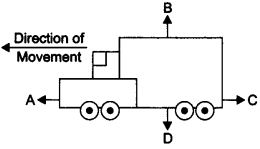Ans: The forces A, B, C and D acting on the truck are:
A → driving force
B → reacting force
C → frictional force
D → weight/gravitational force

Ques 1: State the difference in balanced and unbalanced force.
Ans:

 Balanced force Unbalanced force Forces acting on a body from the opposite directions are same. Forces acting on a body from two opposite directions are not same. It does not change the state of rest or of motion of an object. It do change the state of rest or of motion of an object.

Ques 2: What change will force bring in a body?
Ans: Force can bring following changes in the body:
It can change the speed of a body.
It can change the direction of motion of a body,
It can change the shape of the body.

Ques 3: When a motorcar makes a sharp turn at a high speed, we tend to get thrown to one side. Explain why?
Ans: It is due to law of inertia. When we are sitting in car moving in straight line, we tend to continue in our straight-line motion. But when an unbalanced force is applied by the engine to change the direction of motion of the motorcar. We slip to one side of the seat due to the inertia of our body.

Ques 4: Explain why it is dangerous to jump out of a moving bus.
Ans: While moving in a bus our body is in motion. On jumping out of a moving bus our feet touches the ground and come to rest. While the upper part of our body stays in motion and moves forward due to inertia of motion and hence we can fall in forward direction.
Hence, to avoid this we need to run forward in the direction of bus.

Ques 5: Why do fielders pull their hand gradually with the moving ball while holding a catch?
Ans: While catching a. fast moving cricket ball, a fielder on the ground gradually pulls his hands backwards with the moving ball. This is done so that the fielder increases the time during which the high velocity of the moving ball decreases to zero. Thus, the acceleration of the ball is decreased and therefore the impact of catching the fast moving ball is reduced.

Ques 6: In a high jump athletic event, why are athletes made to fall either on a cushioned bed or on a sand bed?
Ans: In a high jump athletic event, athletes are made to fall either on a cushioned bed or on a sand bed so as to increase the time of the athlete’s fall to stop after making the jump. This decreases the rate of change of momentum and hence the force.

Ques 7: How does a karate player breaks a slab of ice with a single blow?
Ans: A karate player applied the blow with large velocity in a very short interval of time on the ice slab which therefore exerts large amount of force on it and suddenly breaks the ice slab.

Ques 8: What is law of conservation of momentum?
Ans: Momentum of two bodies before collision is equal to the momentum after collision.
In an isolated system, the total momentum remain conserved.

Ques 9. Why are roads on mountains inclined inwards at turns?
Ans: A vehicle moving on mountains is in the inertia of motion. At a sudden turn there is a tendency of vehicle to fall off the road due to sudden change in the line of motion hence the roads are inclined inwards so that the vehicle does not fall down the mountain.

Ques 10: For an athletic races why do athletes have a special posture with their right foot resting on a solid supporter?
Ans: Athletes have to run the heats and they rest their foot on a solid supports before start so that during the start of the race the athlete pushes the support with lot of force and this support gives him equal and opposite push to start the race and get a good start to compete for the race.

Ques 11:Why do you think it is necessary to fasten your seat belts while travelling in your vehicle?
Or
How are safety belts helpful in preventing any accidents?
Ans: While we are travelling in a moving car, our body remains in the state of rest with respect to the seat. But when driver applies sudden breaks or stops the car our body tends to continue in the same state of motion because of its inertia. Therefore, this sudden break may cause injury to us by impact or collision. Hence, safety belt exerts a force on our body to make the forward motion slower.

Ques 12: Explain how momentum gets conserved in collision of two bodies.
Ans: Consider two bodies i.e., balls A and B, the mass and initial velocities are mAuA and mBurespectively before collision. The two bodies collide and force is exerted by each body. There is change in their velocities due to collision.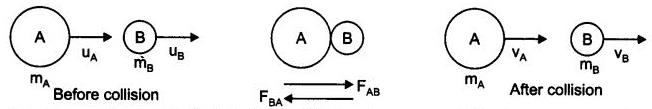The momentum of ball A before collision is mAuA and final momentum is  mAvA. The rate of change of momentum, during the collision for 'A' will be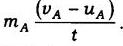Initial momentum of B is mBuand final momentum is mBvB.
Rate of change of momentum of B will be mB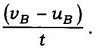According to the third law of motion, the force fAB exerted by ball A on ball B and the force fBA exerted by the ball B on ball A must be equal and opposite to each other. Therefore,
fAB = GBA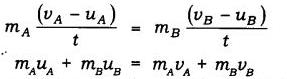∴ (mAuA +mBuB) is the total momentum of the  two balls A and B before collision and (mAvA + mBvB) is their total momentum after collision.
∴ The total momentum of the two balls remains unchanged or conserved provided no other external force acts.

Ques 13: When you kick a football it flies away but when you kick a stone you get huh why?
Ans: This is because stone is heavier than football and heavier objects offer larger inertia.
When we kick a football its mass is less and inertia is also less so force applied by our kick acts on it and hence it shows larger displacement but in case of stone, it has larger mass and offers larger inertia. When we kick (action) the stone it exerts an equal and opposite force (reaction) and hence it hurts the foot.

Ques 14: If a person jumps from a height on a concrete surface he gets hurt. Explain.
Ans: When a person jumps from a height he is in state of inertia of motion. When he suddenly touches the ground he comes to rest in a very short time and hence the force exerted by the hard concrete surface on his body is very high, and the person gets hurt.

Ques 15: What is the relation between Newton’s three laws of motion?
Ans: Newton’s first law explains about the unbalanced force required to bring change in the position of the body.
Second law states/explains about the amount of force required to produce a given acceleration.
And Newton’s third law explains how these forces acting on a body are interrelated.

Ques 16: Give any three examples in daily life which are based on Newton’s third law of motion.
Ans: Three examples based on Newton’s third law are :
(i) Swimming: We push the water backward to move forward.
action – water is pushed behind
reaction – water pushes the swimmer ahead
(ii) Firing gun: A bullet fired from a gun and the gun recoils.
action – gun exerts force on the bullet
reaction – bullet exerts an equal and opposite force on the gun
(ii) Launching of rocket: action – hot gases from the rocket are released reaction – the gases exert upward push to the rocket

Ques 17: A bullet of m.ass 20 g is horizontally fired with a velocity 150 m/s from a pistol of mass 2 kg. What is the
recoil velocity of the pistol?
Ans: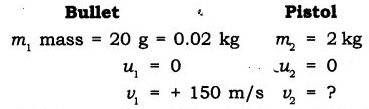Total momentum of the  pistol and bullet before firing, when the gun is at rest
= m1u1 + m2u2
= (0.02 * 0) + (2 * 0)
0 kg m/s
Total momentum of the pistol and bullet after it is fired
= m1v1 + m2v2
= (0.02 kg * 150) + (2 kg * v)
= 3+ 2v
∴ Total momentum after firing = Total momentum before firing
3 + 2v
∴ v = -3/2 = -1.5 m/s

Ques 18: Negative sign indicates that the direction in which the pistol would recoil is opposite to that of bullet.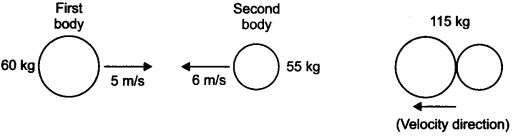Two bodies as shown in the figure collide with each other and join thereafter. With what velocity will they move after combining together?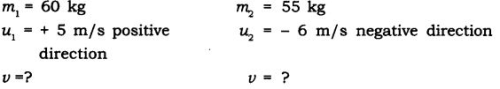Total momentum of two bodies before collision
= m1u+m2u2
= 60 * 5 + 55 * -6
= -30  kg m/s
If v is the velocity  of two joined bodies. After collision, the total momentum will be
= m1v1 + m2v2 (∴ v1 = v2)
= (m1 + m2)v
= (60 + 55) kg * v m/s
= 115 v
∴ According to law of conservation of  momentum
m1u1 + m2u2 = m1v1 +m2v2
- 30 kg m/s = 115v
v = -30/115 = -0.26 m/s
∴ Two bodies will move with velocity  0.26 m/s  in the direction of the second body.

Offer running on EduRev: Apply code STAYHOME200 to get INR 200 off on our premium plan EduRev Infinity!

## Science Class 9

143 videos|224 docs|135 tests

,

,

,

,

,

,

,

,

,

,

,

,

,

,

,

,

,

,

,

,

,

;